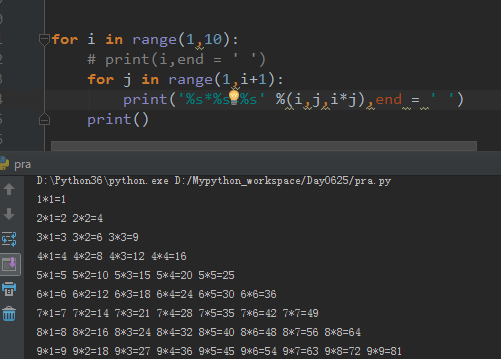# python怎么写乘法表• 爱喝马黛茶的安东尼   2019-10-12 13:39:051338```for i in range(1,10):
# print(i,end = ' ')
for j in range(1,i+1):
print('%s*%s=%s' %(i,j,i*j),end = ' ')
print()```

1.

`for i in range(1,10)`

range（1）：这个取的值是0

range（2）：这个取得值是0，1

range（3）：这个取得值是0，1，2

range（0，3，1）：这个取得值是0，1，2，其中第三个数字1其实就是默认的步长，只是我写出来了，不写也可以。整体写下来默认是从0开始取值的，除非你自己定义数字1，或者2，那就是从1，或者2开始取值。

2.

`for j in range(1,i+1)`

for j in range（1，i+1）

...........

3.

```    print('%s*%s=%s' %(i,j,i*j),end = ' ')
print()```

%s是格式化取值的意思，前面2步都求出来了，就开始打印了%s*%s=%s %（i,j,i*j,end = ' ' ），其中end = “ ”的意思就是在每个计算的结尾处加个空格。主要是排版上好看易于理解一些。然后结果就是：

```1*1=1
2*1=2 2*2=4
3*1=3 3*2=6 3*3=9
4*1=4 4*2=8 4*3=12 4*4=16
5*1=5 5*2=10 5*3=15 5*4=20 5*5=25
.........```